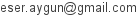14.02.2012 · Print · History · Edit

# L-system Fractal Generator for js1k

For general information on Lindenmayer systems, please see the related Wikipedia article.

The script that I wrote for js1k contest executes given L-system code and generates fractals on the screen. It supports turning (`+` and `−`), drawing (lowercase letters) and push/pop (`[` and `]`) operators.

An L-system code consists of the following lines.

`iters:` n
The number of iterations. The more iterations performed, the more detail is achieved and the more time is spent.
`angle:` Θ
The turning angle in degrees.
`start:` `"`S`"`
The initial axiom. The computation starts with string S.
X`:` `"`Y`"`
A rewrite rule. In each iteration all Xs in the current string are substituted with Ys.

### Examples

#### Fractal of Love by me

```iters: 5
angle: 45
start: "X"
X: "+ff++f++f--f++f++ff"
f: "fff[-ffX][+fX]"
```

#### Koch snowflake

```iters: 5
angle: 60
start: "f--f--f"
f: "f+f--f+f"
```

#### Dragon curve

```iters: 12
angle: 90
start: "fX"
X: "X+Yf+"
Y: "-fX-Y"
```

#### Gosper curve

```iters: 5
angle: 60
start: "Xf"
X: "X+Yf++Yf-fX--fXfX-Yf+"
Y: "-fX+YfYf++Yf+fX--fX-Y"
```

#### Moore curve

```iters: 7
angle: 90
start: "L"
L: "-Rf+LfL+fR-"
R: "+Lf−RfR−fL+"
```

#### Sierpinski triangle

```iters: 8
angle: 60
start: "f"
f: "g-f-g"
g: "f+g+f"
```

#### Fractal plant from Wikipedia

```iters: 6
angle: 25
start: "+++X"
X: "f+[[X]-X]-f[-fX]+X"
f: "ff"
```

#### Fractal plant by Adrian Mariano

```iters: 8
angle: 18
start: "+++++X"
X: "f[+X]f[-X]+X"
f: "ff"
```

#### Another fractal plant by Adrian Mariano

```iters: 4
angle: 22.5
start: "++++f"
f: "ff-[-f+f+f]+[+f-f-f]"
```

### User's Discoveries

#### Swiss Cross by Forrest Oliphant

```iters: 6
angle: 90
start: "x-ff-x"
x: "xf+fx-ff-xf+fx"
```